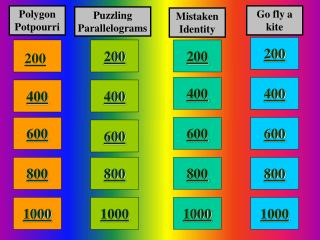# 200 - PowerPoint PPT PresentationDownload Presentation200

200
Download Presentation## 200

- - - - - - - - - - - - - - - - - - - - - - - - - - - E N D - - - - - - - - - - - - - - - - - - - - - - - - - - -
##### Presentation Transcript

1. Polygon Potpourri Go fly a kite Puzzling Parallelograms Mistaken Identity 200 200 200 200 400 400 400 400 600 600 600 600 800 800 800 800 1000 1000 1000 1000

2. Row 1, Col 1 What is concave ? Not Convex.

3. 1,2 What are parallelograms? Being a quadrilateral with both pairs of opposites sides makes any figure a member of this not so exclusive club.

4. 1,3 What is a rhombus? Often mistaken for one of my relatives, I am this type of figure.

5. 1,4 What is a kite? Spanish for “cometa” this quadrilateral has two pairs of consecutive congruent sides, but the opposite side lengths are not congruent.

6. 2,1 What is similar? Figures that share a resemblance. Also figures that are the same shape but not necessarily the same size.

7. 2,2 What is 116 degrees? The figure is a parallelogram

8. 2,3 What is an rectangle? A convex quadrilateral that has two sets of parallel sides, diagonals that bisect each other, and four right angles.

9. 2,4 What is 13 units? Using the Pythagorean theorem with the right triangles formed by the kite’s diagonals would allow us to find the length TU.

10. 3,1 What is an equilateral triangle? There is a much more “normal” way to say equiangular, equilateral, convex and nine sided.

11. 3,2 What is “they bisect each other”? This important quality is a characteristic of the diagonals of quadrilaterals that are also parallelograms.

12. 3,3 What are squares? I could see how you were confused awhile ago, but yes I am both rectangle, and rhombus. No apology necessary.

13. 3,4 What is 88 degrees? The measure of angle K in this kite.

14. 4,1 What is the midsegment of the trapezoid? Parallel to each base, this length is as long the average of the bases in any trapezoid.

15. 4,2 What is a kite? • Of the following I am definitively not a parallelogram. • Rhombus • Kite • Square • Rectangle

16. 4,3 What is an isosceles trapezoid? I’m often called my brother’s name but don’t fall into that “trap” in addition to having parallel bases my legs are also congruent.

17. 4,4 What is 125 degrees? The measure of angle K in this Kite.

18. 5,1 What is (n-2) x 180 P.I.A.T.? By sub-dividing convex polygons into triangles a mathematician was able to come up with this theorem for finding the interior angle measures for a polygon.

19. 5,2 What is 1? This value of ‘x’ makes the quadrilateral a parallelogram.

20. 5,3 What is a square? It appears as thought I am composed of four isosceles right triangles.

21. 5,4 What are 120 degrees (x2), 40 degrees, and 80 degrees? The angle measures of a kite where the four principle interior angles are 3x, 3x, x, and 2x .